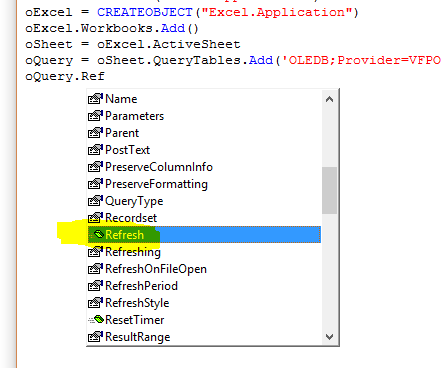# Foxpro and ExcelIt is very possible to automate spreadsheets from Visual Foxpro using VBA/ COM automation. Here I wil document the parts I need.

### Connecting to Excel

``````oExcel = CreateObject("Excel.Application")
if vartype(oExcel) != "O"
* could not instantiate Excel object
* show an error message here
return .F.
endif``````

I use my own library (JLIB) containing a ReturnObject function that fetches errors. Using that function my code looks like this:

``````PUBLIC goX && global Excel object

IF InitiateExcel()
* Code here
ELSE
? [Error initiating Excel automation]
ENDIF

PROCEDURE InitiateExcel
goX = ReturnObject([excel.application])
RETURN (VARTYPE(goX)=[O])
ENDPROC ``````

``oWorkbook = oExcel.Application.Workbooks.Open("C:\temp\test.xls")``

### Create a new blank spreadsheet

``oWorkbook = oExcel.Application.Workbooks.Add()``

### Create a new spreadsheet from a template

``oWorkbook = oExcel.Application.Workbooks.Add("C:\temp\template.xlt")``

### Sheets collection/ objects

``````oWorkbook.sheets.count && returns the number of tabs/ sheets
oSheet = oWorkbook.sheets(1) && get an object to control the sheet
? oSheet.name && show the sheet name``````

### Tables

`A table is called a ListObject in Excel/ Microsoft terminology`
``````oSheet.ListObjects.count && gives the number of tables on the sheet
oTable = oSheet.ListObjects(1) && get an object to control the table
? oTable.ListRows.count && displays the number of data rows in the table
oTable.DataBodyRange.delete && deletes al rows. Error (crash) if there are no rows!``````

### Working with table rows

Adding a table row needs several steps: 1. Add the row and keep the reference to it. 2. Add the column data 1 by 1:

``````oRow = oTable.ListRows.Add && you have a new row and a reference to it
oRow.Range(1) = Date(2020,7,6) && Add a date in the first column``````

### Using named cells

``````? oSheet.Range("ProductionDate").value && show value of cell named ProductionDate
oSheet.Range("TotalPages").value = 13``````

### Saving and closing

The save method is in the workbook object. And you can quit from the Excel application object:

``````oSheet.SaveAs([x:\reports\report.xlsx]) && Save
oExcel.Quit && quit Excel``````

The Quit only works on this Excel application you’ve initiated. So existing opened Excel sheets are untouched (remain opened).